# Pierre de Fermat (1601 - 1665)

From A Short Account of the History of Mathematics' (4th edition, 1908) by W. W. Rouse Ball.

While Descartes was laying the foundations of analytical geometry, the same subject was occupying the attention of another and not less distinguished Frenchman. This was Fermat. Pierre de Fermat, who was born near Montauban in 1601, and died at Castres on January 12, 1665, was the son of a leather-merchant; he was educated at home; in 1631 he obtained the post of councillor for the local parliament at Toulouse, and he discharged the duties of the office with scrupulous accuracy and fidelity. There, devoting most of his leisure to mathematics, he spent the remainder of his life - a life which, but for a somewhat acrimonious dispute with Descartes on the validity of certain analysis used by the latter, was unruffled by any event which calls for special notice. The dispute was chiefly due to the obscurity of Descartes, but the tact and courtesy of Fermat brought it to a friendly conclusion. Fermat was a good scholar, and amused himself by conjecturally restoring the work of Apollonius on plane loci.

Except a few isolated papers, Fermat published nothing in his lifetime, and gave no systematic exposition of his methods. Some of the most striking of his results were found after his death on loose sheets of paper or written in the margins of works which he had read and annotated, and are unaccompanied by any proof. It is thus somewhat difficult to estimate the dates and originality of his work. He was constitutionally modest and retiring, and does not seem to have intended his papers to be published. It is probable that he revised his notes as occasion required, and that his published works represent the final form of his researches, and therefore cannot be dated much earlier than 1660. I shall consider separately (i) his investigations in the theory of numbers; (ii) his use in geometry of analysis and of infinitesimals; and (iii) his method for treating questions of probability.

(i) The theory of numbers appears to have been the favourite study of Fermat. He prepared an edition of Diophantus, and the notes and comments thereon contain numerous theorems of considerable elegance. Most of the proofs of Fermat are lost, and it is possible that some of them were not rigorous - an induction by analogy and the intuition of genius sufficing to lead him to correct results. The following examples will illustrate these investigations.

(a) If p be a prime and a be prime to p then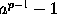is divisible by p, that is,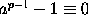(mod p). A proof of this, first given by Euler, is well known. A more general theorem is that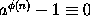(mod n), where a is prime to n and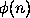is the number of integers less than n and prime to it.

(b) An odd prime can be expressed as the difference of two square integers in one and only one way. Fermat's proof is as follows. Let n be the prime, and suppose it equal to x² - y², that is, to (x + y)(x - y). Now, by hypothesis, the only integral factors of n are n and unity, hence x + y = n and x - y = 1. Solving these equations we get x = ½ (n + 1) and y = ½ (n - 1).

(c) He gave a proof of the statement made by Diophantus that the sum of the squares of two integers cannot be of the form 4n - 1; and he added a corollary which I take to mean that it is impossible that the product of a square and a prime of the form 4n - 1 [even if multiplied by a number prime to the latter], can be either a square or the sum of two squares. For example, 44 is a multiple of 11 (which is of the form 4 × 3 - 1) by 4, hence it cannot be expressed as the sum of two squares. He also stated that a number of the form a² + b², where a is prime to b, cannot be divided by a prime of the form 4n - 1.

(d) Every prime of the form 4n + 1 is expressible, and that in one way only, as the sum of two squares. This problem was first solved by Euler, who shewed that a number of the form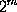(4n + 1) can be always expressed as the sum of two squares.

(e) If a, b, c, be integers, such that a² + b² = c², then ab cannot be a square. Lagrange gave a solution of this.

(f) The determination of a number x such that x²n + 1 may be a square, where n is a given integer which is not a square. Lagrange gave a solution of this.

(g) There is only one integral solution of the equation x² + 2 = y³; and there are only two integral solutions of the equation x² + 4 = y³. The required solutions are evidently for the first equation x = 5, and for the second equation x = 2 and x = 11. This question was issued as a challenge to the English mathematicians Wallis and Digby.

(h) No integral values of x, y, z can be found to satisfy the equation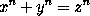; if n be an integer greater than 2. This proposition has acquired extraordinary celebrity from the fact that no general demonstration of it has been given, but there is no reason to doubt that it is true.

Probably Fermat discovered its truth first for the case n = 3, and then for the case n = 4. His proof for the former of these cases is lost, but that for the latter is extant, and a similar proof for the case of n = 3 was given by Euler. These proofs depend on shewing that, if three integral values of x, y, z can be found which satisfy the equation, then it will be possible to find three other and smaller integers which also satisfy it: in this way, finally, we shew that the equation must be satisfied by three values which obviously do not satisfy it. Thus no integral solution is possible. It would seem that this method is inapplicable to any cases except those of n = 3 and n = 4.

Fermat's discovery of the general theorem was made later. A proof can be given on the assumption that a number can be resolved into the product of powers of primes in one and only one way. The assumption has been made by some writers; it is true of real numbers, but it is not necessarily true of every complex number. It is possible that Fermat made some erroneous supposition, but, on the whole, it seems more likely that he discovered a rigorous demonstration.

In 1823 Legendre obtained a proof for the case of n = 5; in 1832 Lejeune Dirichlet gave one for n = 14, and in 1840 Lamé and Lebesgue gave proofs for n = 7. The proposition appears to be true universally, and in 1849 Kummer, by means of ideal primes, proved it to be so for all numbers except those (if any) which satisfy three conditions. It is not certain whether any number can be found to satisfy these conditions, but there is no number less than 100 which does so. The proof is complicated and difficult, and there can be no doubt is based on considerations unknown to Fermat. I may add that, to prove the truth of the proposition, when n is greater than 4 obviously it is sufficient to confine ourselves to cases when n is a prime, and the first step in Kummer's demonstration is to shew that one of the numbers x, y, z must be divisible by n.

The following extracts, from a letter now in the university library at Leyden, will give an idea of Fermat's methods; the letter is undated, but it would appear that, at the time Fermat wrote it, he had proved the proposition (h) above only for the case when n = 3.

Je ne m'en servis au commencement qe pour demontrer les propositions negatives, comme par exemple, qu'il n'y a aucu nombre moindre de l'unité qu'un multiple de 3 qui soit composé d'un quarré et du triple d'un autre quarré. Qu'il n'y a aucun triangle rectangle de nombres dont l'aire soit un nombre quarré. La preuve se fait par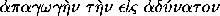en cette manière. S'il y auoit aucun triangle rectangle en nombres entiers, qui eust son aire esgale à un quarré, il y auroit un autre triangle moindre que celuy la qui auroit la mesme proprieté. S'il y en auoit un second moindre que le premier qui eust la mesme proprieté il y en auroit par un pareil raisonnement un troisieme moindre que ce second qui auroit la mesme proprieté et enfin un quatrieme, un cinquieme etc. a l'infini en descendant. Or est il qu'estant donné un nombre il n'y en a point infinis en descendant moindres que celuy la, j'entens parler tousjours des nombres entiers. D'ou on conclud qu'il est donc impossible qu'il y ait aucun triangle rectange dont l'aire soit quarré. Vide foliu post sequens....

Je fus longtemps sans pouvour appliquer ma methode aux questions affirmatives, parce que le tour et le biais pour y venir est beaucoup plus malaisé que celuy dont je me sers aux negatives. De sorte que lors qu'il me falut demonstrer que tout nombre premier qui surpasse de l'unité un multiple de 4, est composé de deux quarrez je me treuvay en belle peine. Mais enfin une meditation diverses fois reiterée me donna les lumieres qui me manquoient. Et les questions affirmatives passerent par ma methods a l'ayde de quelques nouveaux principes qu'il y fallust joindre par necessité. Ce progres de mon raisonnement en ces questions affirmatives estoit tel. Si un nombre premier pris a discretion qui surpasse de l'unité un multiple de 4 n'est point composé de deux quarrez il y aura un nombre premier de mesme nature moindre que le donné; et ensuite un troisieme encore moindre, etc. en descendant a l'infini jusques a ce que vous arriviez au nombre 5, qui est le moindre de tous ceux de cette nature, lequel il s'en suivroit n'estre pas composé de deux quarrez, ce qu'il est pourtant d'ou on doit inferer par la deduction a l'impossible que tous ceux de cette nature sont par consequent composez de 2 quarrez.

Il y a infinies questions de cette espece. Mais il y en a quelques autres que demandent de nouveaux principes pour y appliquer la descente, et la recherche en est quelques fois si mal aisée, qu'on n'y peut venir qu'avec une peine extreme. Telle est la question suivante que Bachet sur Diophante avoüe n'avoir jamais peu demonstrer, sur le suject de laquelle Mr. Descartes fait dans une de ses lettres la mesme declaration, jusques la qu'il confesse qu'il la juge si difficile, qu'il ne voit point de voye pour la resoudre. Tout nombre est quarré, ou composé de deux, de trois, ou de quatre quarrez. Je l'ay enfin rangée sous ma methode et je demonstre que si un nombre donné n'estoit point de cette nature il y en auroit un moindre que ne le seroit par non plus, puis un troisieme moindre que le second etc. a l'infini, d'ou l'on infere que tous les nombres sont de cette nature....

J'ay ensuit consideré questions que bien que negatives ne restent pas de recevoir tres-grande difficulté, la methods pour y pratiquer la descente estant tout a fait diverse des precedentes comme il sera aisé d'espouver. Telles sont les suivantes. Il n'y a aucun cube divisible en deux cubes. Il n'y a qu'un seul quarré en entiers que augmenté du binaire fasse un cube, ledit quarré est 25. Il n'y a que deux quarrez en entiers lesquels augmentés de 4 fassent cube, lesdits quarrez sont 4 et 121....

Apres avoir couru toutes ces questions la plupart de diverses (sic) nature et de differente façon de demonstrer, j'ay passé a l'invention des regles generales pour resoudre les equations simples et doubles de Diophante. On propose par exemple 2 quarr. + 7957 esgaux a un quarré (hoc est 2xx + 7967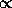quadr.) J'ay une regle generale pour resoudre cette equation si elle est possible, on decouvrir son impossibilité. Et ainsi en tous les cas et en tous nombres tant des quarrez que des unitez. On propose cette equation double 2x + 3 et 3x + 5 esgaux chaucon a un quarré. Bachet se glorifie en ses commentaires sur Diophante d'avoir trouvé une regle en deux cas particuliers. Je me donne generale en toute sorte de cas. Et determine par regle si elle est possible ou non....

Voila sommairement le conte de mes recherches sur le sujet des nombres. Je ne l'ay escrit que parce que j'apprehende que le loisir d'estendre et de mettre au long toutes ces demonstrations et ces methodes me manquera. En tout cas cette indication seruira aux sçauants pour trouver d'eux mesmes ce que je n'estens point, principlement si Mr. de Carcaui et Frenicle leur font part de quelques demonstrations par la descente que je leur ay envoyees sur le suject de quelques propositions negatives. Et peut estre la posterité me scaure gré de luy avoir fait connoistre que les anciens n'ont pas tout sceu, et cette relation pourra passer dans l'esprit de ceux qui viendront apres moy pour traditio lampadis ad filios, comme parle le grand Chancelier d'Angleterre, suivant le sentiment et la devise duquel j'adjousteray, multi pertransibunt et augebitur scientia.

(ii) I next proceed to mention Fermat's use in geometry of analysis and of infinitesimals. It would seem from his correspondence that he had thought out the principles of analytical geometry for himself before reading Descartes's Géométrie, and had realised that from the equation, or, as he calls it, the `specific property,'' of a curve all its properties could be deduced. His extant papers on geometry deal, however, mainly with the application of infinitesimals to the determination of the tangents to curves, to the quadrature of curves, and to questions of maxima and minima; probably these papers are a revision of his original manuscripts (which he destroyed), and were written about 1663, but there is no doubt that he was in possession of the general idea of his method for finding maxima and minima as early as 1628 or 1629.

He obtained the subtangent to the ellipse, cycloid, cissoid, conchoid, and quadratrix by making the ordinates of the curve and a straight line the same for two points whose abscissae were x and x - e; but there is nothing to indicate that he was aware that the process was general, it is probable that he never separated it, so to speak, from the symbols of the particular problem he was considering. The first definite statement of the method was due to Barrow, and was published in 1669.

Fermat also obtained the areas of parabolas and hyperbolas of any order, and determined the centres of mass of a few simple laminae and of a paraboloid of revolution. As an example of his method of solving these questions I will quote his solution of the problem to find the area between the parabola y³ = p x², the axis of x, and the line x = a. He says that, if the several ordinates of the points for which x is equal to a, a(1 - e), a(1 - e)²,... be drawn, then the area will be split into a number of little rectangles whose areas are respectively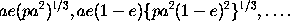The sum of these is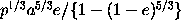; and by a subsidiary proposition (for he was not acquainted with the binomial theorem) he finds the limit of this, when e vanishes, to be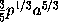. The theorems last mentioned were published only after his death; and probably they were not written till after he had read the works of Cavalieri and Wallis.

Kepler had remarked that the values of a function immediately adjacent to and on either side of a maximum (or minimum) value must be equal. Fermat applied this principle to a few examples. Thus, to find the maximum value of x(a - x), his method is essentially equivalent to taking a consecutive value of x, namely x - e where e is very small, and putting x(a - x) = (x - e)(a - x + e). Simplifying, and ultimately putting e = 0, we get x = ½. This value of x makes the given expression a maximum.

(iii) Fermat must share with Pascal the honour of having founded the theory of probabilities. I have already mentioned the problem proposed to Pascal, and which he communicated to Fermat, and have there given Pascal's solution. Fermat's solution depends on the theory of combinations, and will be sufficiently illustrated by the following example, the substance of which is taken from a letter dated August 24, 1654, which occurs in the correspondence with Pascal. Fermat discusses the case of two players, A and B, where A wants two points to win and B three points. Then the game will be certainly decided in the course of four trials. Take the letters a and b, and write down all the combinations that can be formed of four letters. These combinations are 16 in number, namely, aaaa, aaab, aaba, aabb; abaa, abab, abba, abbb; baaa, baab, baba, babb; bbaa, bbab, bbba, bbbb. Now every combination in which a occurs twice or oftener represents a case favourable to A, and every combination in which b occurs three times or oftener represents a case favourable to B. Thus, on counting them, it will be found that there are 11 cases favourable to A, and 5 cases favourable to B; and since these cases are all equally likely, A's chance of winning the game is to B's chance as 11 is to 5.

The only other problem on this subject which, as far as I know, attracted the attention of Fermat was also proposed to him by Pascal, and was as follows. A person undertakes to throw a six with a die in eight throws; supposing him to have made three throws without success, what portion of the stake should he be allowed to take on condition of giving up his fourth throw? Fermat's reasoning is as follows. The chance of success is 1/6, so that he should be allowed to take 1/6 of the stake on condition of giving up his throw. But if we wish to estimate the value of the fourth throw before any throw is made, then the first throw is worth 1/6 of the stake; the second is worth 1/6 of what remains, that is 5/36 of the stake; the third throw is worth 1/6 of what now remains, that is, 25/216 of the stake; the fourth throw is worth 1/6 of what now remains, that is, 125/1296 of the stake.

Fermat does not seem to have carried the matter much further, but his correspondence with Pascal shows that his views on the fundamental principles of the subject were accurate: those of Pascal were not altogether correct.

Fermat's reputation is quite unique in the history of science. The problems on numbers which he had proposed long defied all efforts to solve them, and many of them yielded only to the skill of Euler. One still remains unsolved. This extraordinary achievement has overshadowed his other work, but in fact it is all of the highest order of excellence, and we can only regret that he thought fit to write so little.

This page is included in a collection of mathematical biographies taken from A Short Account of the History of Mathematics by W. W. Rouse Ball (4th Edition, 1908).

Transcribed by

D.R. Wilkins
(dwilkins@maths.tcd.ie)
School of Mathematics
Trinity College, Dublin